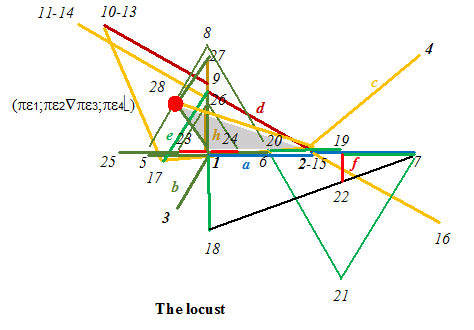# The locust

The usual paradigm for Euclidean geometry is that one can do every construction with a ruler and a compass. The obvious construction is that of a triangle from three given side lengths. However it turns  out that it is sufficient in Euclidean geometry if one requests that one can construct any isosceles triangle (a triangle with two legs of same length).

Based on the parallel axiom one can then construct every triangle from its three given side lengths. One starts from points 1 and 2 for the base line a , point 3 gives the length of another side b by its distance to point 1 , point 4 gives the length of the third side c by its distance to point 2. For obvious reasons the construction for point 28 is called locustition .